# 相机标定（Camera calibration）原理、步骤

## 相机标定（Camera calibration）原理、步骤

author@jason_ql（lql0716）
http://blog.csdn.net/lql0716

• 在图像测量过程以及机器视觉应用中，为确定空间物体表面某点的三维几何位置与其在图像中对应点之间的相互关系，必须建立相机成像的几何模型，这些几何模型参数就是相机参数。在大多数条件下这些参数必须通过实验与计算才能得到，这个求解参数的过程就称之为相机标定（或摄像机标定）。无论是在图像测量或者机器视觉应用中，相机参数的标定都是非常关键的环节，其标定结果的精度及算法的稳定性直接影响相机工作产生结果的准确性。因此，做好相机标定是做好后续工作的前提，提高标定精度是科研工作的重点所在。

• 常用术语
内参矩阵: Intrinsic Matrix
焦距: Focal Length
主点: Principal Point
切向畸变: Tangential Distortion
旋转矩阵: Rotation Matrices
平移向量: Translation Vectors
平均重投影误差: Mean Reprojection Error
重投影误差: Reprojection Errors
重投影点: Reprojected Points

# 1、坐标系的转换

## 1.3 世界坐标系转换为相机坐标系

xcyczc1=[R0t1]xwywzw1

## 1.4 像素坐标系、图像坐标系

• 图1：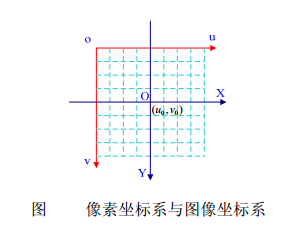• 像素坐标系（pixel coordinate）
如图1，像素坐标系uov$uov$是一个二维直角坐标系，反映了相机CCD/CMOS芯片中像素的排列情况。原点o$o$位于图像的左上角，u$u$轴、v$v$轴分别于像面的两边平行。像素坐标系中坐标轴的单位是像素（整数）

像素坐标系不利于坐标变换，因此需要建立图像坐标系XOY$XOY$其坐标轴的单位通常为毫米（mm），原点是相机光轴与相面的交点（称为主点），即图像的中心点，X$X$轴、Y$Y$轴分别与u$u$轴、v$v$轴平行。故两个坐标系实际是平移关系，即可以通过平移就可得到。

• 图像坐标系转换为像素坐标系

uv1=1/dX0001/dY0u0v01XY1

## 1.5 针孔成像原理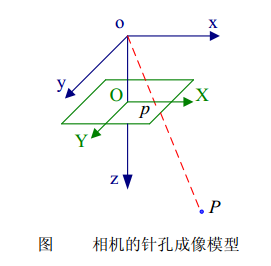sXY1=f000f0001000xyz1

## 1.6 世界坐标系转换为像素坐标系

suv1=1/dX0001/dY0u0v01f000f0001000[R0t1]xwywzw1=αx000αy0u0v01000[R0t1]xwywzw1=M1M2Xw=MXw

# 2 相机内参与畸变参数

• 参看1.6节所述

## 2.2 畸变参数

• 畸变参数(distortion parameters)

畸变（distortion）是对直线投影（rectilinear projection）的一种偏移。

简单来说直线投影是场景内的一条直线投影到图片上也保持为一条直线。

畸变简单来说就是一条直线投影到图片上不能保持为一条直线了，这是一种光学畸变（optical aberration）,可能由于摄像机镜头的原因。

• 畸变一般可以分为：径向畸变、切向畸变

1、径向畸变来自于透镜形状
2、切向畸变来自于整个摄像机的组装过程

畸变还有其他类型的畸变，但是没有径向畸变、切向畸变显著

• 畸变图示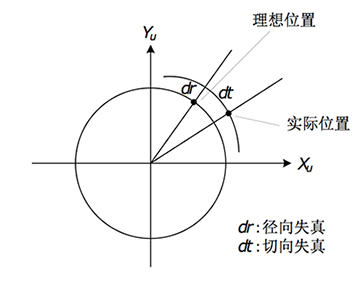• 径向畸变

实际摄像机的透镜总是在成像仪的边缘产生显著的畸变，这种现象来源于“筒形”或“鱼眼”的影响。

如下图，光线在原理透镜中心的地方比靠近中心的地方更加弯曲。对于常用的普通透镜来说，这种现象更加严重。筒形畸变在便宜的网络摄像机中非常厉害，但在高端摄像机中不明显，因为这些透镜系统做了很多消除径向畸变的工作。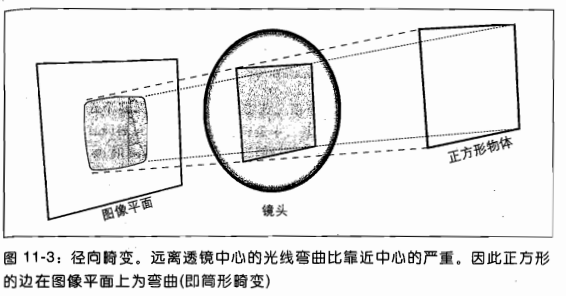对于径向畸变，成像仪中心（光学中心）的畸变为0，随着向边缘移动，畸变越来越严重。

• 径向畸变包括：枕形畸变、桶形畸变

• 切向畸变

• 切向畸变是由于透镜制造上的缺陷使得透镜本身与图像平面不平行而产生的。

• 切向畸变可分为：薄透镜畸变、离心畸变

• 切向畸变图示：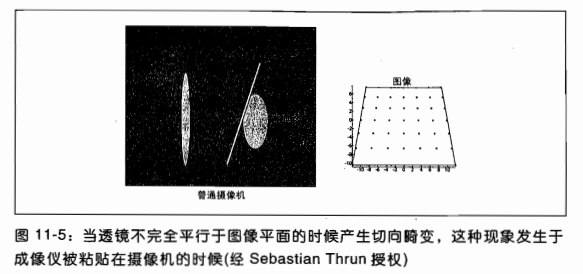### 2.2.1 opencv中的畸变模型

• 径向畸变模型：

δxr=x(k1r2+k2r4+k3r6+K)

δyr=y(k1r2+k2r4+k3r6+K)

• 切向畸变模型：

δxd=2p1xy+p2(r2+2x2)+K

δyd=2p1(r2+2y2)+2p2xy+K

• 理想坐标(x,y)$(x^{'}, y^{'})$与真实坐标(x,y)$(x,y)$

x=x+δxr+δxd

y=y+δyr+δyd

[xy]=(1+k1r2+k2r4+k3r6)[xy]+[2p1xy+p2(r2+2x2)2p1(r2+2y2)+2p2xy]

### 2.2.2 张氏标定中的畸变模型

• 张正友的方法只考虑了径向畸变，没有考虑切向畸变

• 模型：

• 理想情况下(没有畸变)图片的像素坐标为：

(u,v)

• 真实的像素坐标为：

(u¯,v¯)

• 真实坐标与理想坐标的关系式（泰勒展开）：
(x,y)$(x,y)$ and (x¯,y¯)$(\bar{x},\bar{y})$ are the ideal (distortion-free) and real (distorted) normalized image coordinates.

x¯=x+x[k1(x2+y2)+k2(x2+y2)2]

y¯=y+y[k1(x2+y2)+k2(x2+y2)2]

其中k1$k_1$k2$k_2$是径向畸变系数(coefficients of the radial distortion)。

像素坐标表示为：

u¯=u+(uu0)[k1(x2+y2)+k2(x2+y2)2]

v¯=v+(vv0)[k1(x2+y2)+k2(x2+y2)2]

即：

[(uu0)(x2+y2)(vv0)(x2+y2)(uu0)(x2+y2)2(vv0)(x2+y2)][k1k2]=[u¯uv¯v]

### 2.2.3 Tasi畸变模型

• Tasi畸变模型同张氏畸变模型类似，也只考虑了径向畸变

## 2.3 相机标定

• 相机标定步骤：
1、打印一张棋盘格，把它贴在一个平面上，作为标定物。
2、通过调整标定物或摄像机的方向，为标定物拍摄一些不同方向的照片。
3、从照片中提取棋盘格角点。
4、估算理想无畸变的情况下，五个内参和六个外参。
5、应用最小二乘法估算实际存在径向畸变下的畸变系数。
6、极大似然法，优化估计，提升估计精度。

# 3 Matlab相机标定

• 打开matlab，找到“Camera Calibrator”并打开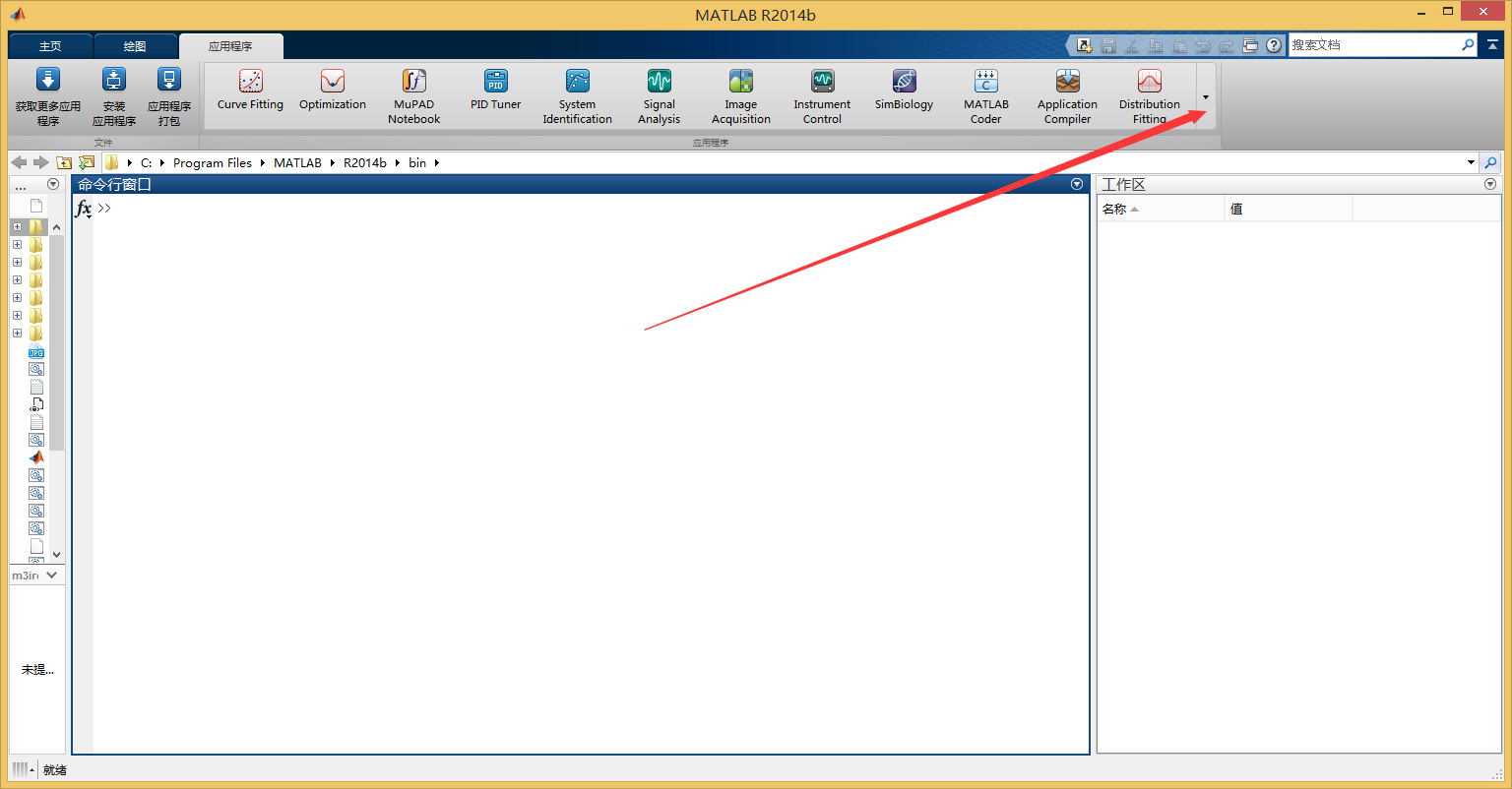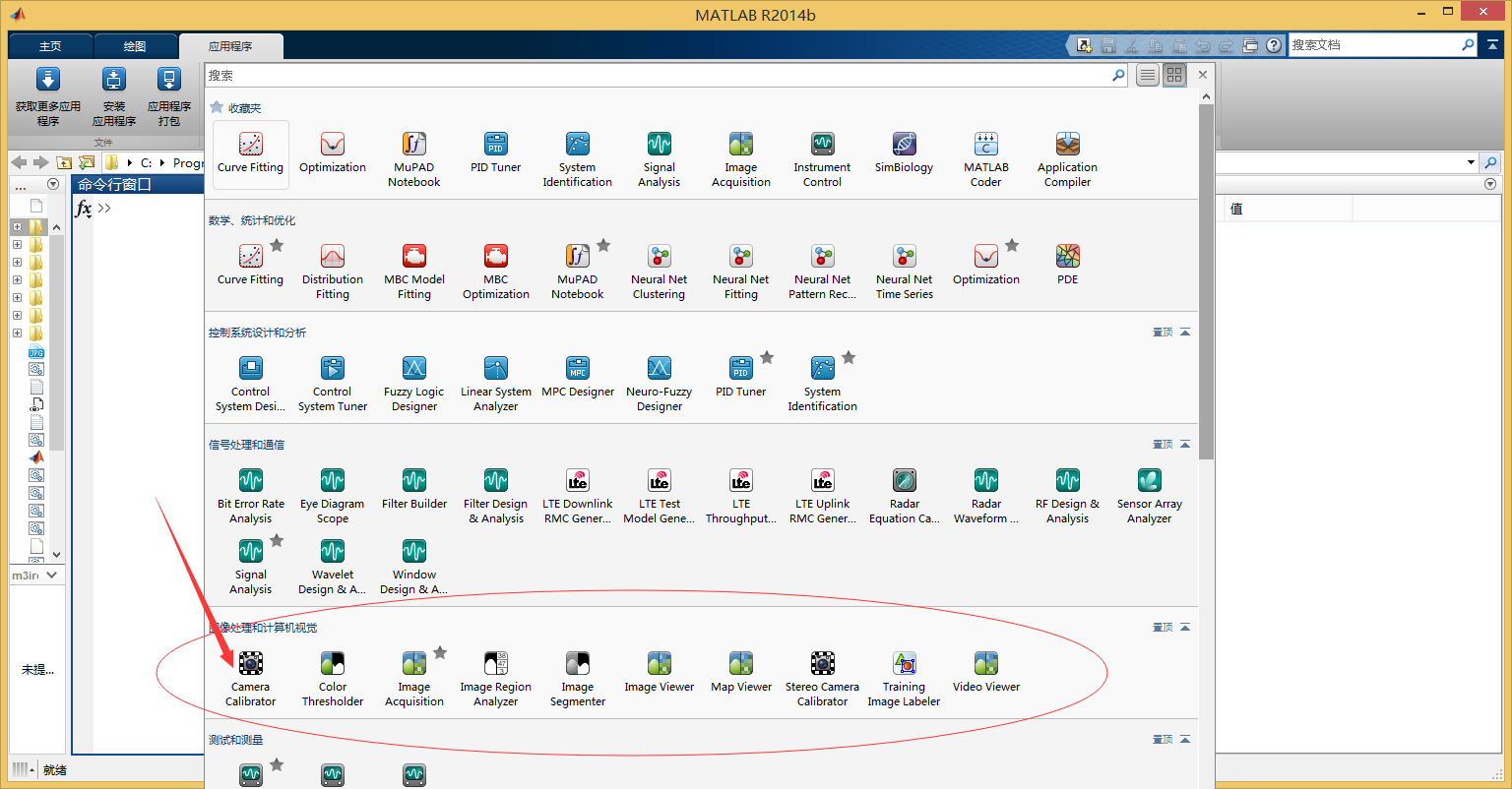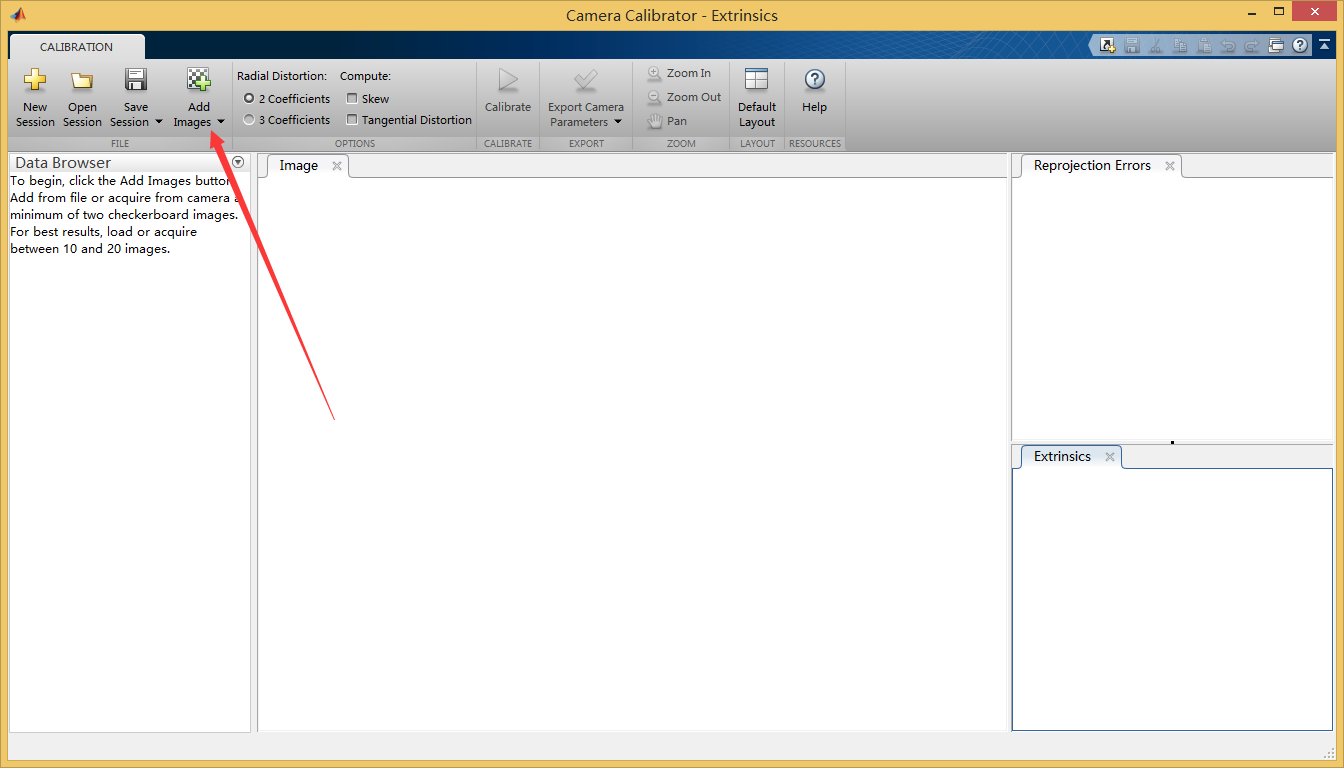• 添加图片之后，会有如下提示，设置棋盘格的实际大小之后，点击ok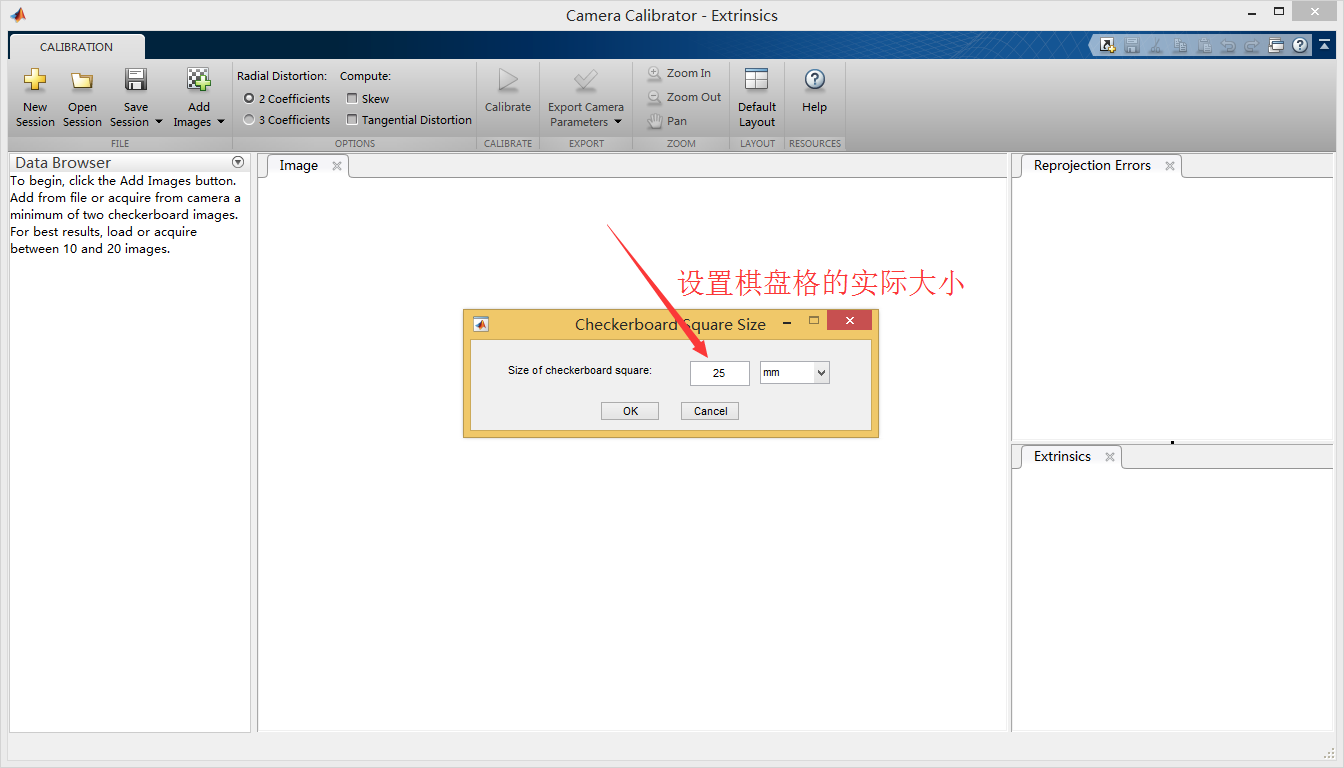• 点击“Calibrate”开始计算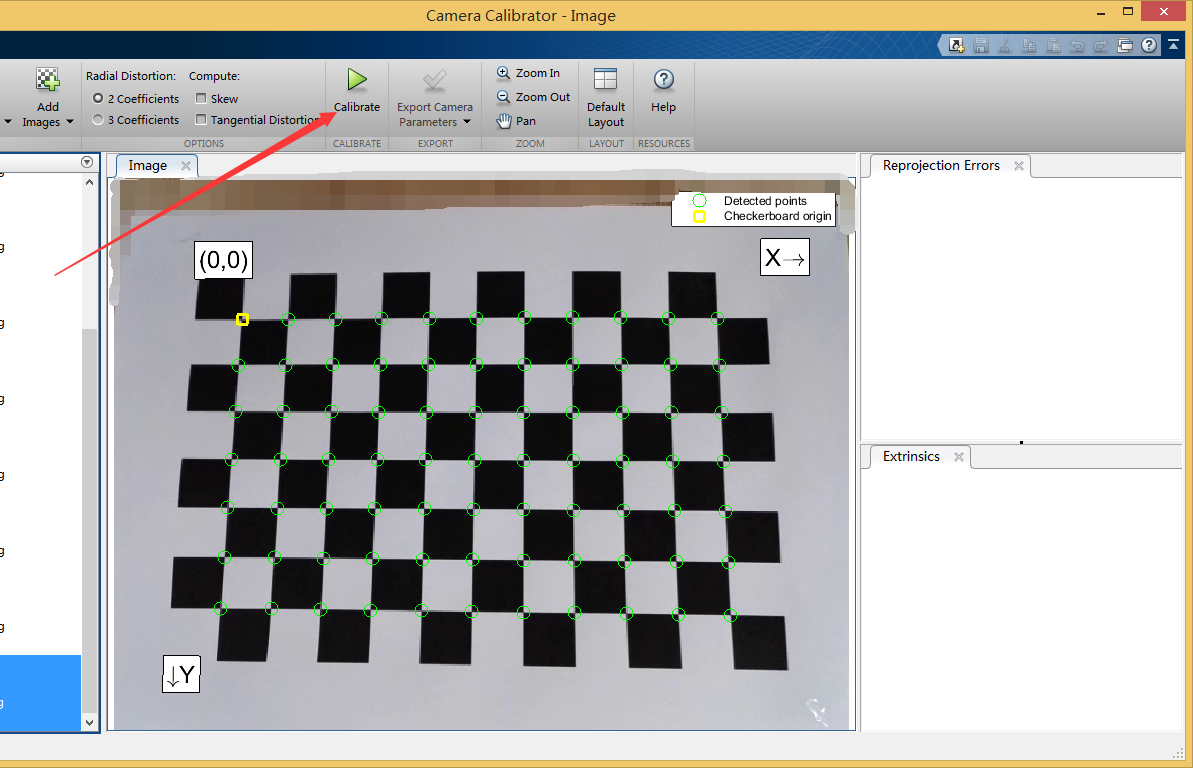• 点击“Export Camera Parameters”，输出到matlab命令窗口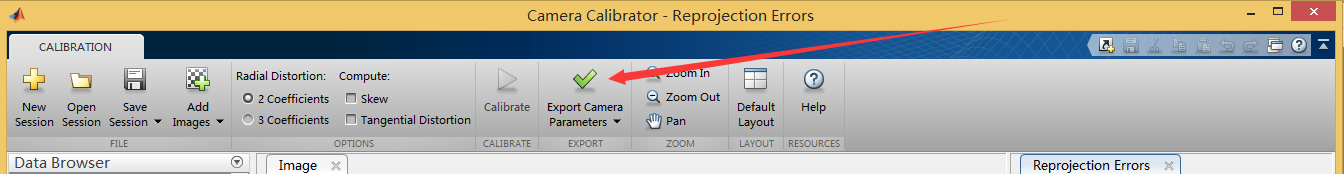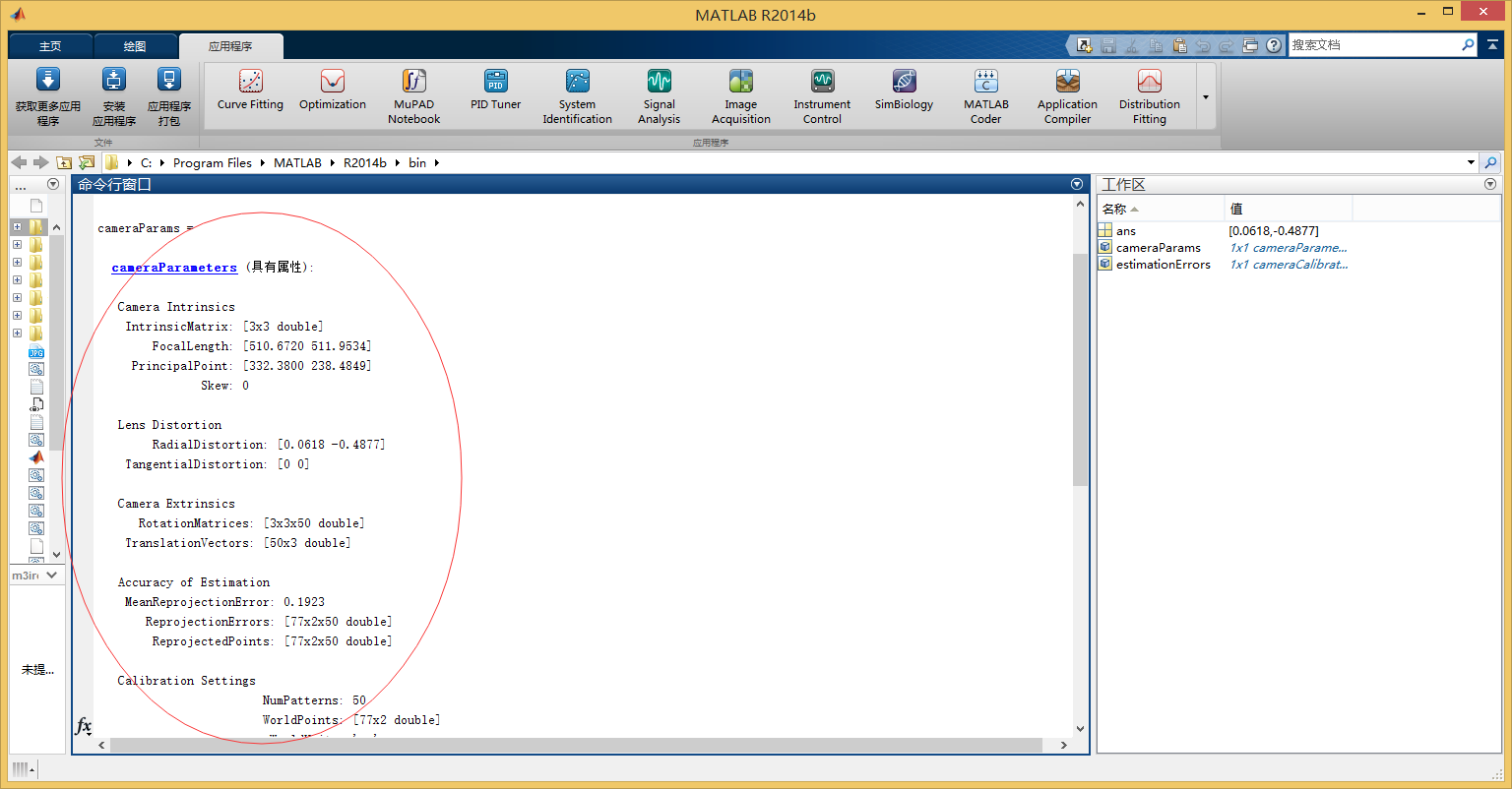• 导出的数据如下：
cameraParams =

cameraParameters (具有属性):

Camera Intrinsics
IntrinsicMatrix: [3x3 double]
FocalLength: [510.6720 511.9534]
PrincipalPoint: [332.3800 238.4849]
Skew: 0

Lens Distortion
TangentialDistortion: [0 0]

Camera Extrinsics
RotationMatrices: [3x3x50 double]
TranslationVectors: [50x3 double]

Accuracy of Estimation
MeanReprojectionError: 0.1923
ReprojectionErrors: [77x2x50 double]
ReprojectedPoints: [77x2x50 double]

Calibration Settings
NumPatterns: 50
WorldPoints: [77x2 double]
WorldUnits: 'mm'
EstimateSkew: 0
EstimateTangentialDistortion: 0

estimationErrors =

cameraCalibrationErrors (具有属性):

IntrinsicsErrors: [1x1 intrinsicsEstimationErrors]
ExtrinsicsErrors: [1x1 extrinsicsEstimationErrors]
• 在命令窗口输入以下命令即可获得内参矩阵和径向畸变：
>> cameraParams.IntrinsicMatrix

ans =

510.6720         0         0
0  511.9534         0
332.3800  238.4849    1.0000

0.0618   -0.4877